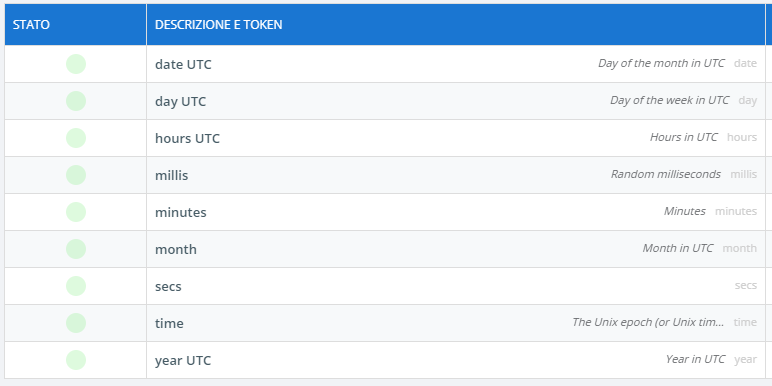# Calculate a signal on an hourly basis

Let's consider for example the electricity supply costs, we want a virtual signal that varies costs depending on time.

In Databoom Starter Pack device there are some time signals that can be used to calculate virtual signals on hourly basis.

• If Databoom Starter Pack is not available in devices list, check device subscription in public devices list (Devices -> Public in side menu)It's therefore possible to create a virtual signal that uses the hours UTC signal as variable, along with your energy signal.

With the formula

`(x0>=0 AND x0<6) ? y0 * 0.2 : ((x0>=6 AND x0<15) ? y0 * 0.4 : ((x0>=15 AND x0<19) ? y0 * 0.6 : y0 * 0.3))`

it's possible to consider different costs depending on hours UTC signal vaue, in this case the x0 variable. In the example we have 4 time slots:

• from 00:00 to 05:59 with a 0.2 cost
• from 06:00 to 14:59 with a 0.4 cost
• from 15:00 to 18:59 with a 0.6 cost
• from 19:00 to 23:59, with a 0.3 cost

It's important to consider timezone while creating time slots, hours UTC logs the hour value in UTC timezone.